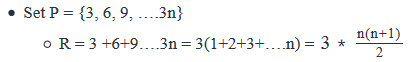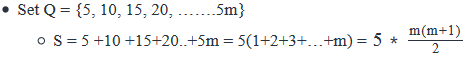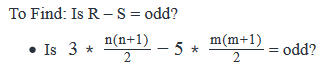## GMAT QuestionDeeksha Raina Raina Dec 15, 2020
I did something different. Please tell me where I might have gone wrong.

P = 3,6,9...3n
Q= 5,10,15...5m

R = 3+6+9..3n
= 3(1+2+3..n) = 3*n(n+1)/2

S = 5*m(m+1)/2 .

We have to chk of R- S is odd.
i.e 3*n(n+1)/2 - 5*m(m+1)/2 is Odd.

Now Statement I -- m is odd and n is even.

If m is Odd then m+1 will be Even.
If n is Even n+1 will be Odd.

Therefore m(m+1) will be Odd*Even which is Even.
And n(n+1) will be Even*Odd which is Even.

So both terms will be divisible by 2 hence R-S will be 3 - 5 = -2 which isn't Odd.

Statement 2 says -- m is 4x+3 ( Which is Odd). Hence m+1 will be 4x+4 (Which is Even).
And n is 2x (Which is Even) . Hence n+1 will be 2x+1 ( Which is Odd.

Therefore again, the product will be even as seen in Statement I hence the answer will also be Even.

Therefore we prove that R-S is not Odd from both statement alone.

Is this wrong?MANDAPATI LAKSHMI AJAY SAI Jan 27, 2019
Steps 1 & 2: Understand Question and Draw Inferences• Difference of two integers will be odd, only if one of the numbers is odd.
• Let’s see when R and S will be odd:
• The even-odd nature of R will depend on the values of n and n + 1. Since n and n+ 1 are consecutive integers, only one of them can be even.
• Now, in the expression of R as n(n+1) is divided by 2, for R to be even, either of n or n + 1 should be a multiple of 4, i.e. they should be of the form 4k, where k is a positive integer. Hence, following cases arise:
• n is of the form 4k, i.e. n = 4k OR
• n+1 is of the form 4k, i.e. n + 1 = 4k
• So, n = 4k – 1 = 4(k -1) + 4 -3 = 4(k-1) + 3
• So, we can say that R will be even if n is of the form 4k or 4k + 3. For all the other cases, R will be odd
• Since the expression of S is similar to R, we can say that S will be even if m is of the form 4k or 4k + 3
• So, we need to look for values of m and n in the statements.
Step 3: Analyze Statement 1 independently
(1) m is odd and n is even
• As we do not know if m or n is of the form of 4k or 4k + 3, we cannot say, if R and S are odd or even.

Step 4: Analyze Statement 2 independently
(2) m can be expressed in the form of 4x +3 and n can be expressed in the form of 2x, where x is a positive integer
• As m can be expressed in the form of 4x + 3, S will be even.
• As n can be expressed in the form of 2x, n may be expressed in the form of 4x or may not be expressed in the form of 4x.
• So, R may be odd or R may be even.
• If R is odd,  R – S = odd
• If R is even, R – S = even.
• Hence, we cannot say for sure if R – S is odd.

Step 5: Analyze Both Statements Together (if needed)
(1) From statement-1, we know that  is odd and n is even
(2) From statement-2, we know that R may be even or odd and S is even
• Combining both the statements does not tell us anything about the even-odd nature of R, hence we cannot say for sure if R – S = odd.